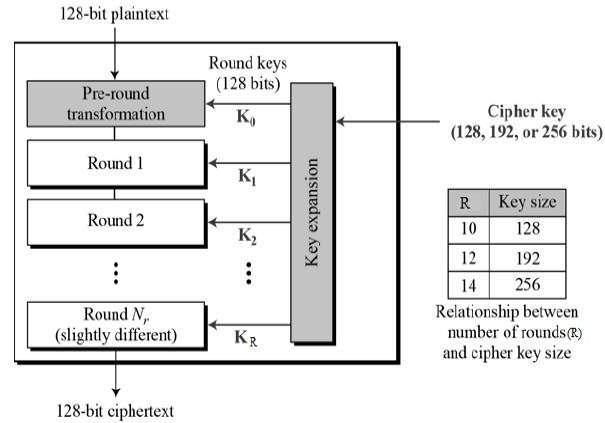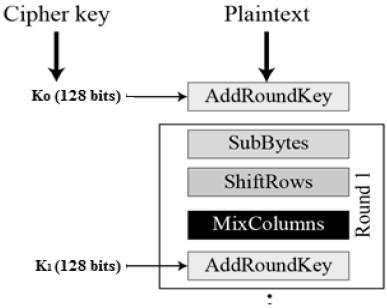The more popular and widely adopted symmetric encryption algorithm likely to be encountered nowadays is the Advanced Encryption Standard (AES). It is found at least six time faster than triple DES.

A replacement for DES was needed as its key size was too small. With increasing computing power, it was considered vulnerable against exhaustive key search attack. Triple DES was designed to overcome this drawback but it was found slow.

The features of AES are as follows −

• Symmetric key symmetric block cipher
• 128-bit data, 128/192/256-bit keys
• Stronger and faster than Triple-DES
• Provide full specification and design details
• Software implementable in C and Java

## Operation of AES

AES is an iterative rather than Feistel cipher. It is based on ‘substitution–permutation network’. It comprises of a series of linked operations, some of which involve replacing inputs by specific outputs (substitutions) and others involve shuffling bits around (permutations).

Interestingly, AES performs all its computations on bytes rather than bits. Hence, AES treats the 128 bits of a plaintext block as 16 bytes. These 16 bytes are arranged in four columns and four rows for processing as a matrix −

Unlike DES, the number of rounds in AES is variable and depends on the length of the key. AES uses 10 rounds for 128-bit keys, 12 rounds for 192-bit keys and 14 rounds for 256-bit keys. Each of these rounds uses a different 128-bit round key, which is calculated from the original AES key.

The schematic of AES structure is given in the following illustration −## Encryption Process

Here, we restrict to description of a typical round of AES encryption. Each round comprise of four sub-processes. The first round process is depicted below −### Byte Substitution (SubBytes)

The 16 input bytes are substituted by looking up a fixed table (S-box) given in design. The result is in a matrix of four rows and four columns.

### Shiftrows

Each of the four rows of the matrix is shifted to the left. Any entries that ‘fall off’ are re-inserted on the right side of row. Shift is carried out as follows −

• First row is not shifted.

• Second row is shifted one (byte) position to the left.

• Third row is shifted two positions to the left.

• Fourth row is shifted three positions to the left.

• The result is a new matrix consisting of the same 16 bytes but shifted with respect to each other.

### MixColumns

Each column of four bytes is now transformed using a special mathematical function. This function takes as input the four bytes of one column and outputs four completely new bytes, which replace the original column. The result is another new matrix consisting of 16 new bytes. It should be noted that this step is not performed in the last round.

The 16 bytes of the matrix are now considered as 128 bits and are XORed to the 128 bits of the round key. If this is the last round then the output is the ciphertext. Otherwise, the resulting 128 bits are interpreted as 16 bytes and we begin another similar round.

## Decryption Process

The process of decryption of an AES ciphertext is similar to the encryption process in the reverse order. Each round consists of the four processes conducted in the reverse order −

• Mix columns
• Shift rows
• Byte substitution

Since sub-processes in each round are in reverse manner, unlike for a Feistel Cipher, the encryption and decryption algorithms needs to be separately implemented, although they are very closely related.

## AES Analysis

In present day cryptography, AES is widely adopted and supported in both hardware and software. Till date, no practical cryptanalytic attacks against AES has been discovered. Additionally, AES has built-in flexibility of key length, which allows a degree of ‘future-proofing’ against progress in the ability to perform exhaustive key searches.

However, just as for DES, the AES security is assured only if it is correctly implemented and good key management is employed.